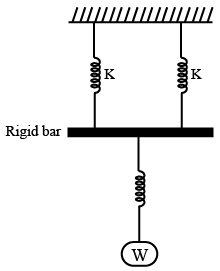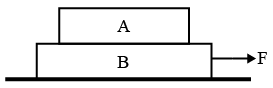# ISRO Scientist or Engineer Mechanical 2018

Instructions

For the following questions answer them individually

Question 71

# ‘If X is a normal variable with mean $$\mu$$ and standard deviation $$\sigma$$, then the mean and standard deviation of the variable $$Z = \frac{X - \mu}{\sigma}$$ isQuestion 72

# Example for an elliptic partial differential equation isQuestion 73

# The solution of the equation $$y'' + y = 0$$ satisfy the condition $$y(0) = 1, y\left(\frac{\pi}{2}\right) = 2$$, isQuestion 74

# For the spring system given in figure, the equivalent stiffness isQuestion 75

# A round uniformly tapered bar of length L and Young’s modulus E has diameter of ‘a’ at one end and ‘2d’ at the other end. If the bar is pulled by an axial force F, the extension produced will beQuestion 76

# Two blocks A and B of 1 kg each are kept on flat surface, one above other. The coefficient of friction between each contacting pair is 0.2. If the lower block is pulled with a horizontal force F, the minimum value of F for which the slip occurs between the blocks is nearlyQuestion 77

# A solid metal bar of uniform diameter D and length L is hung vertically. If $$\rho$$ is density and E is the Young’s modulus, then the total elongation due to self weight isQuestion 78

# A circular solid disc of uniform thickness 20 mm, radius 400 mm and mass 10 kg, is used as a flywheel. If it rotates at 300 rom, the kinetic energy of the flywheel, in Joules isQuestion 79

# If the length of the cantilever beam is reduced to $$\frac{1}{4}th$$, the natural frequency of the mass M at the end of this cantilever beam of negligible mass will be increased by a factor ofQuestion 80

# The relationship between the linear elastic properties Young’s modulus (E), rigidity modulus (G) and Bulk modulus (K) is given byOR